### Continuity

Ex. No. 3.1

1. Are the following functions continuous on the set of real numbers? Justify your answers:

(i) f(x) = 7 [video]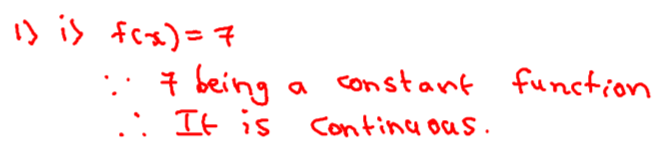(ii) f(x) = e [video]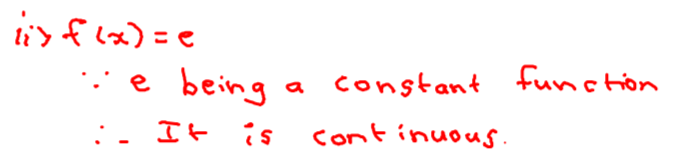(iii) f(x)  = log 19 [video]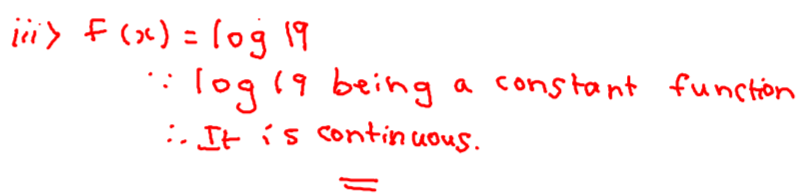(iv) f(x) = 7x4 - 5x3 - 3x + 1 [video]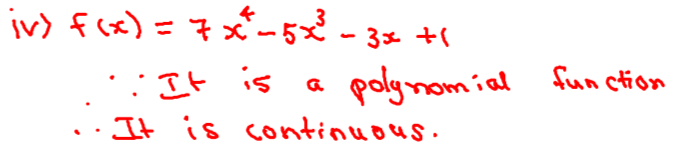(v) g(x) = sin(4x - 3) [video]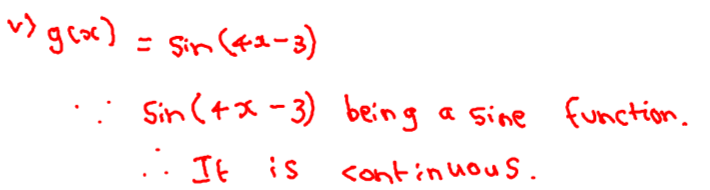(vi) h(x) = (5x2 + 7x + 2) / x3 + x2 + x + 3 [video]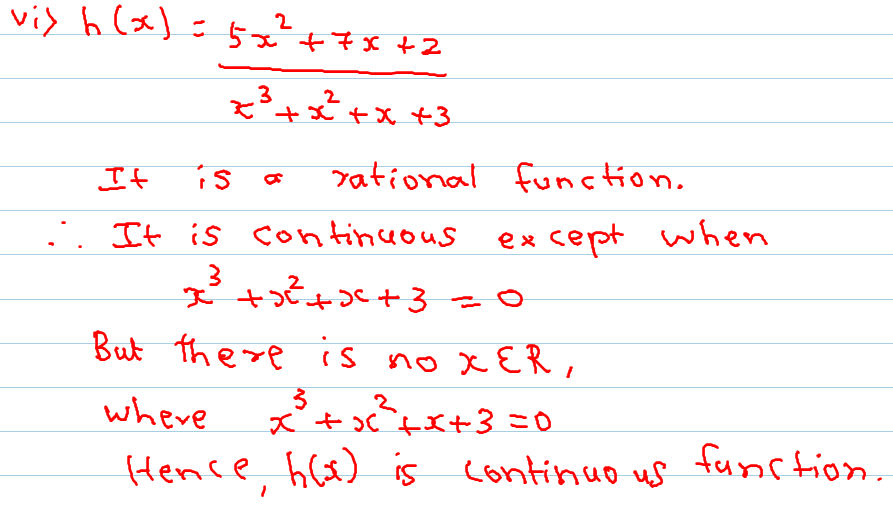vii. g(x) = 13x2 + 16x + 19 [video]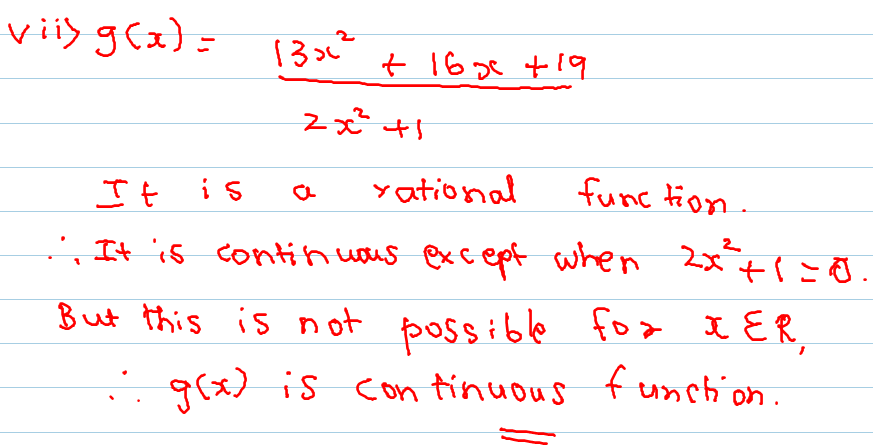viii. f(x) = 5x [video]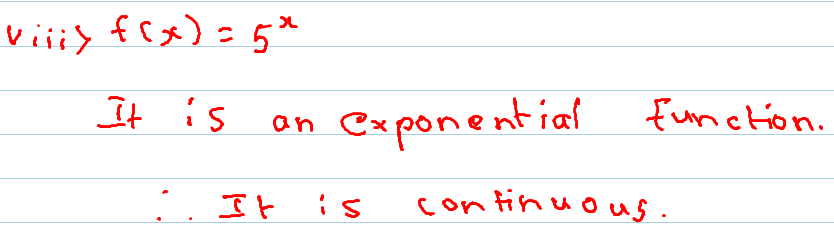ix. f(x) = 32x - 15x [video]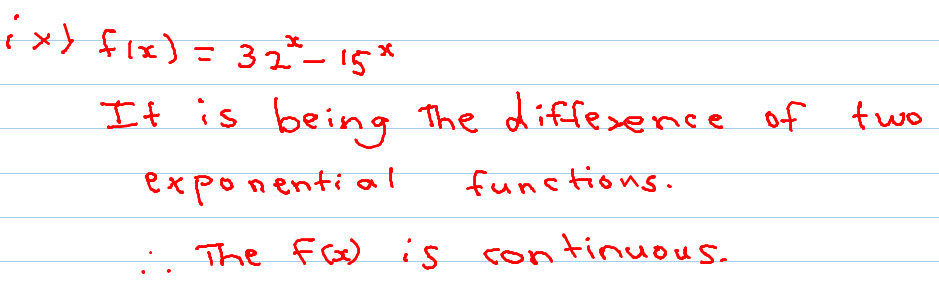x. f(x) = e5x+7  [video]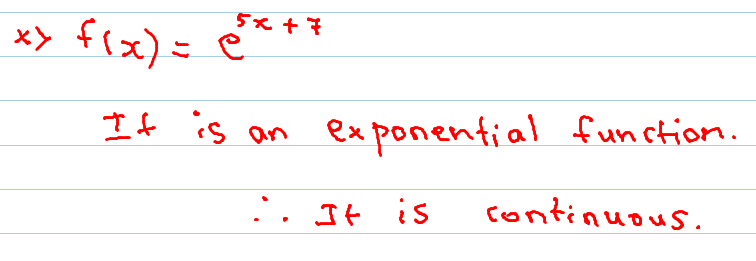### Continuity Ex. no. 3.1 Sum No. 2 Part Three

#### 1 comment:

1.Sir q3 4 5 6 7 8 9 10 bhi hai woh sab kidr hai?